# Frank Solutions for Class 9 Maths Chapter 13 Inequalities in Triangles

Frank Solutions for Class 9 Maths Chapter 13 Inequalities in Triangles improve students’ skills and techniques to solve difficult questions with speed and accuracy. Practising these solutions on a daily basis is the most convenient way to score desired marks in exams. Students who find Maths a complex subject are advised to make use of Frank Solutions as the answers are available in an interactive manner. For in-depth knowledge of the concepts, access Frank Solutions for Class 9 Maths Chapter 13 Inequalities in triangles, from the links mentioned below

Chapter 13 deals with the study of inequality in triangles. This chapter provides formulas and properties of inequalities in triangles which are important from an exam perspective. With the help of these solutions, students can realize the areas of weakness and work on them. These solutions boost problem solving and time management skills among students, irrespective of their intelligence quotient.

## Frank Solutions for Class 9 Maths Chapter 13 Inequalities in Triangles Download PDF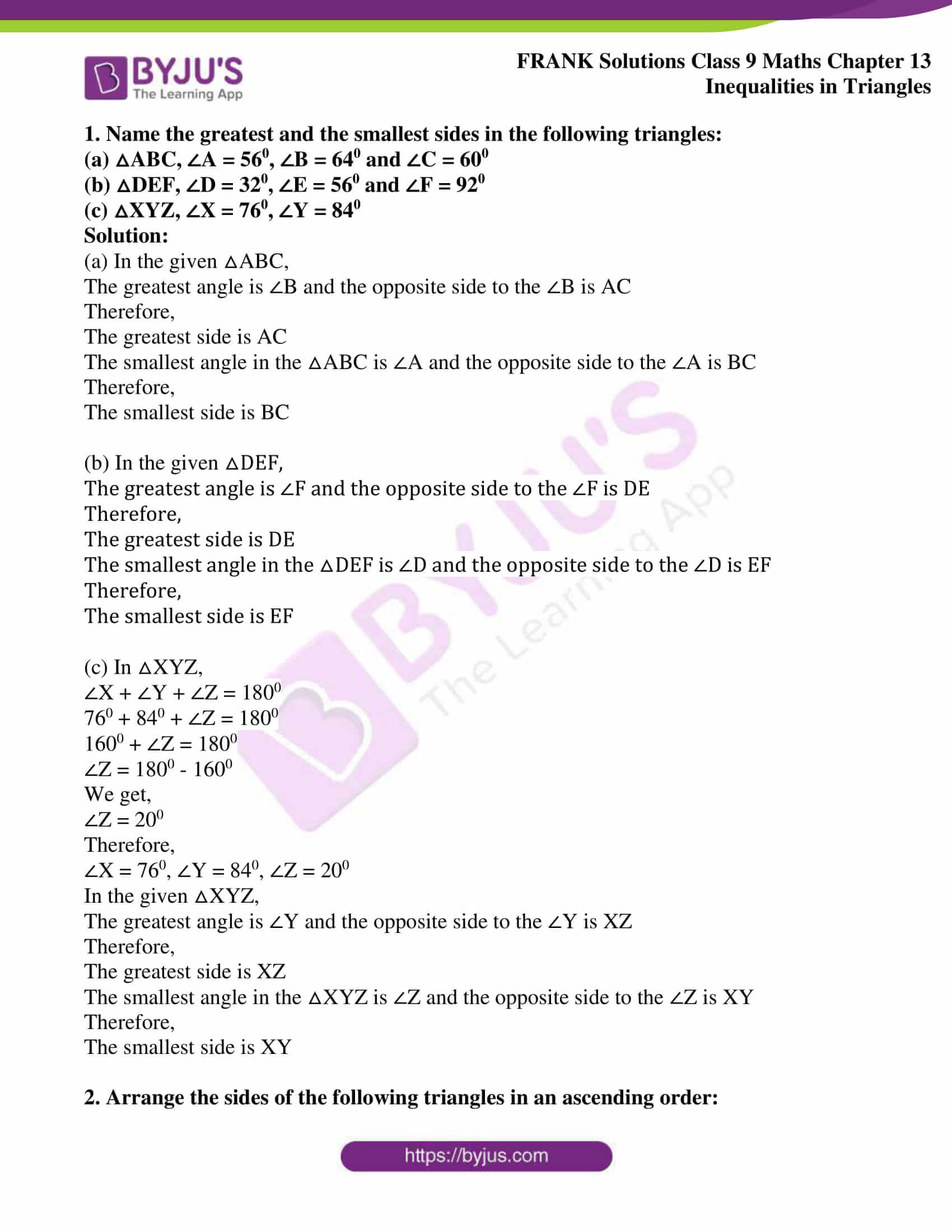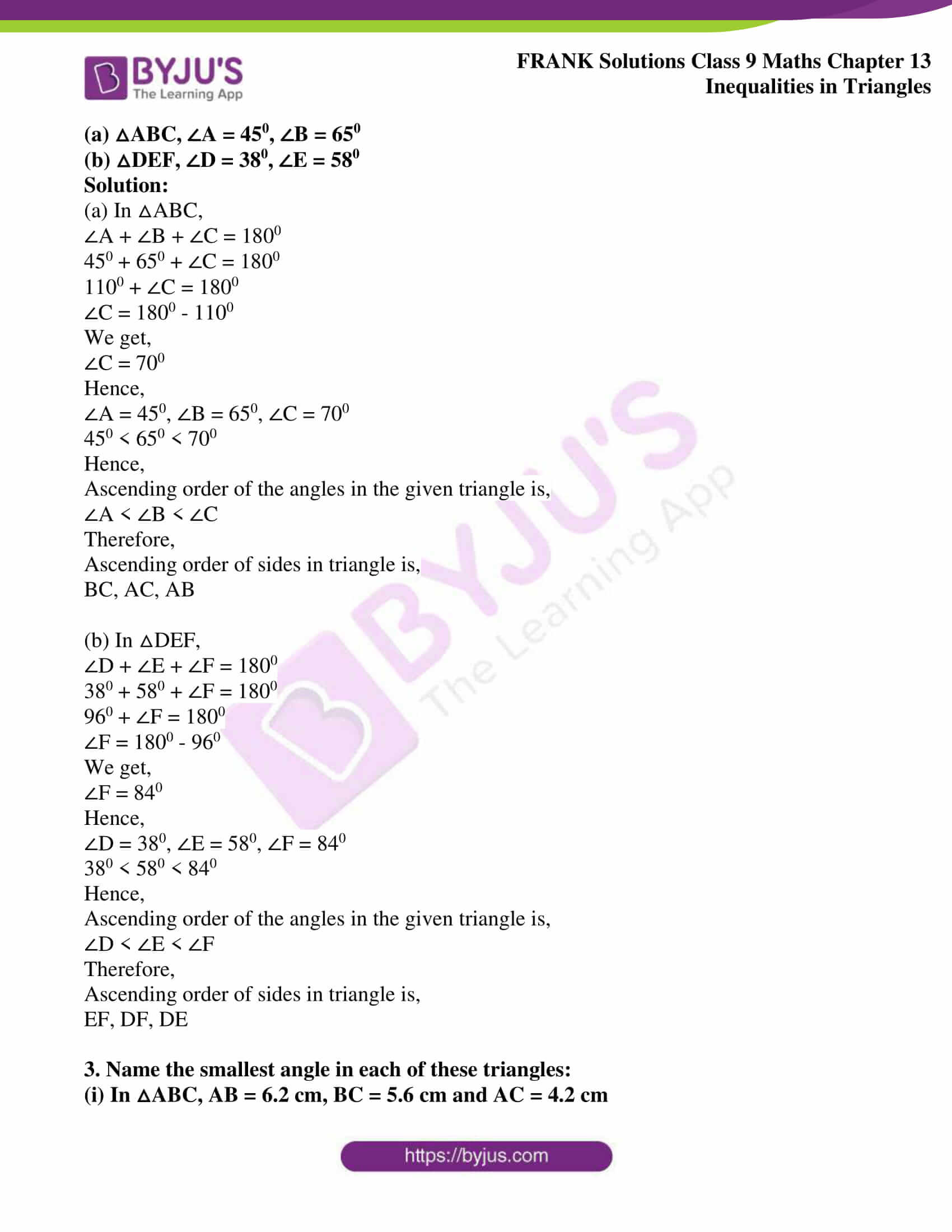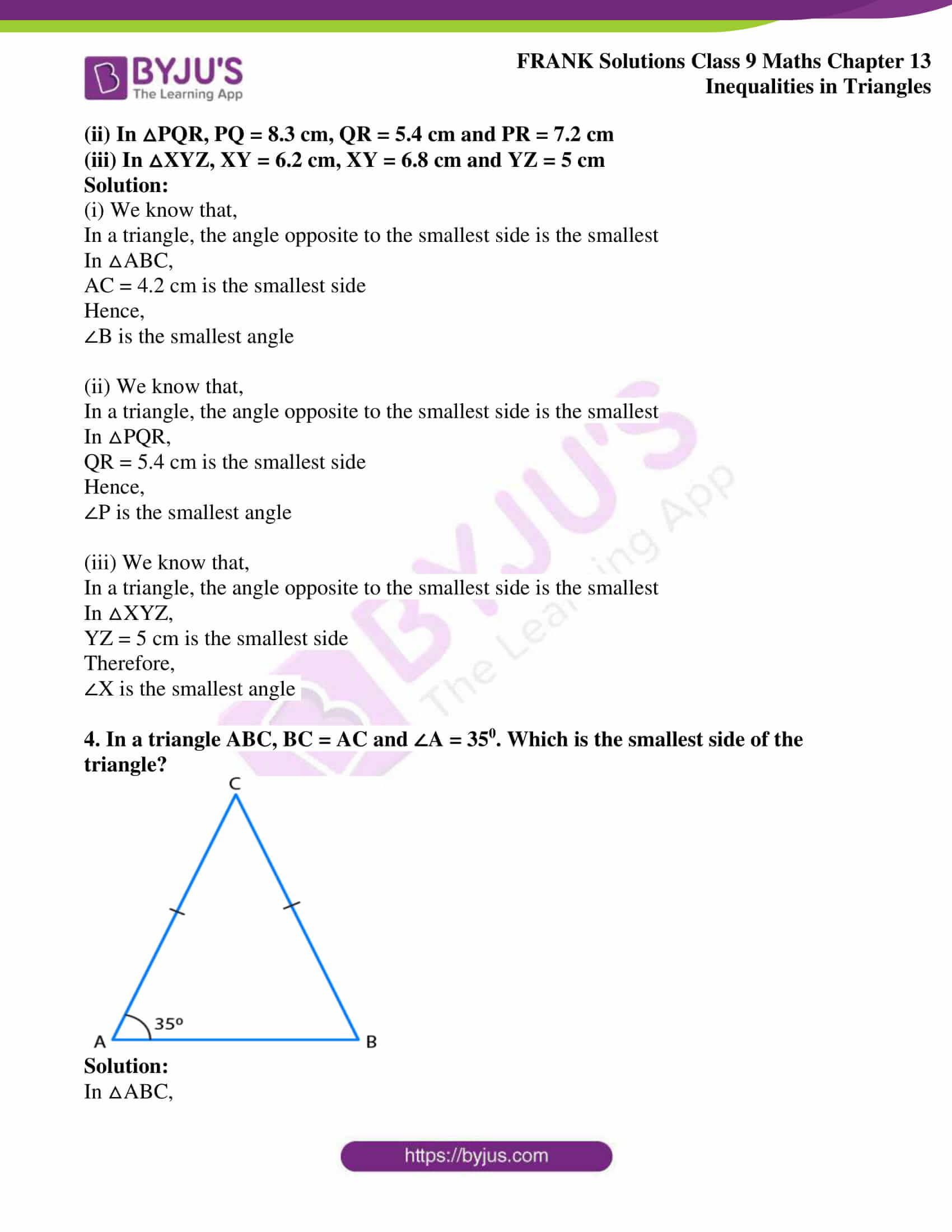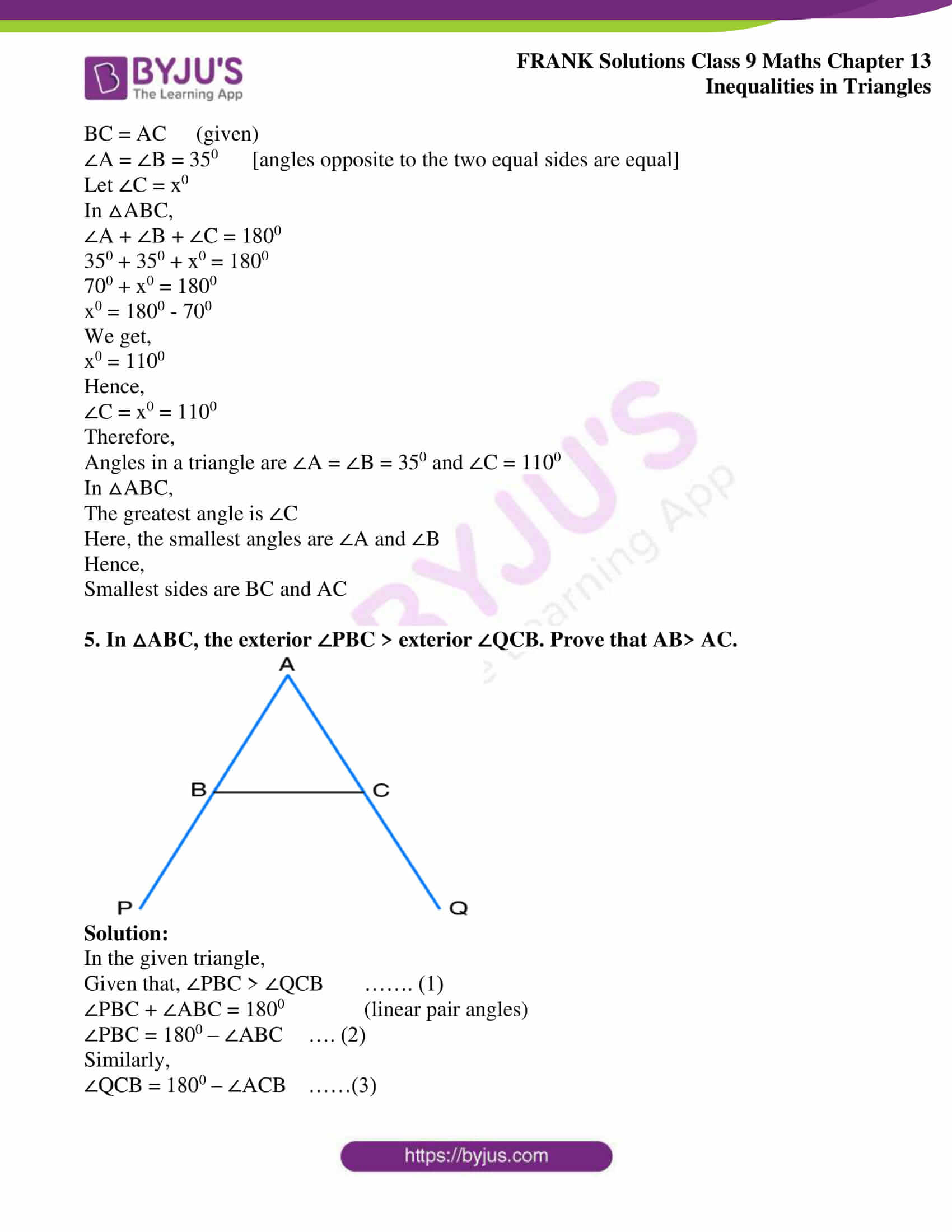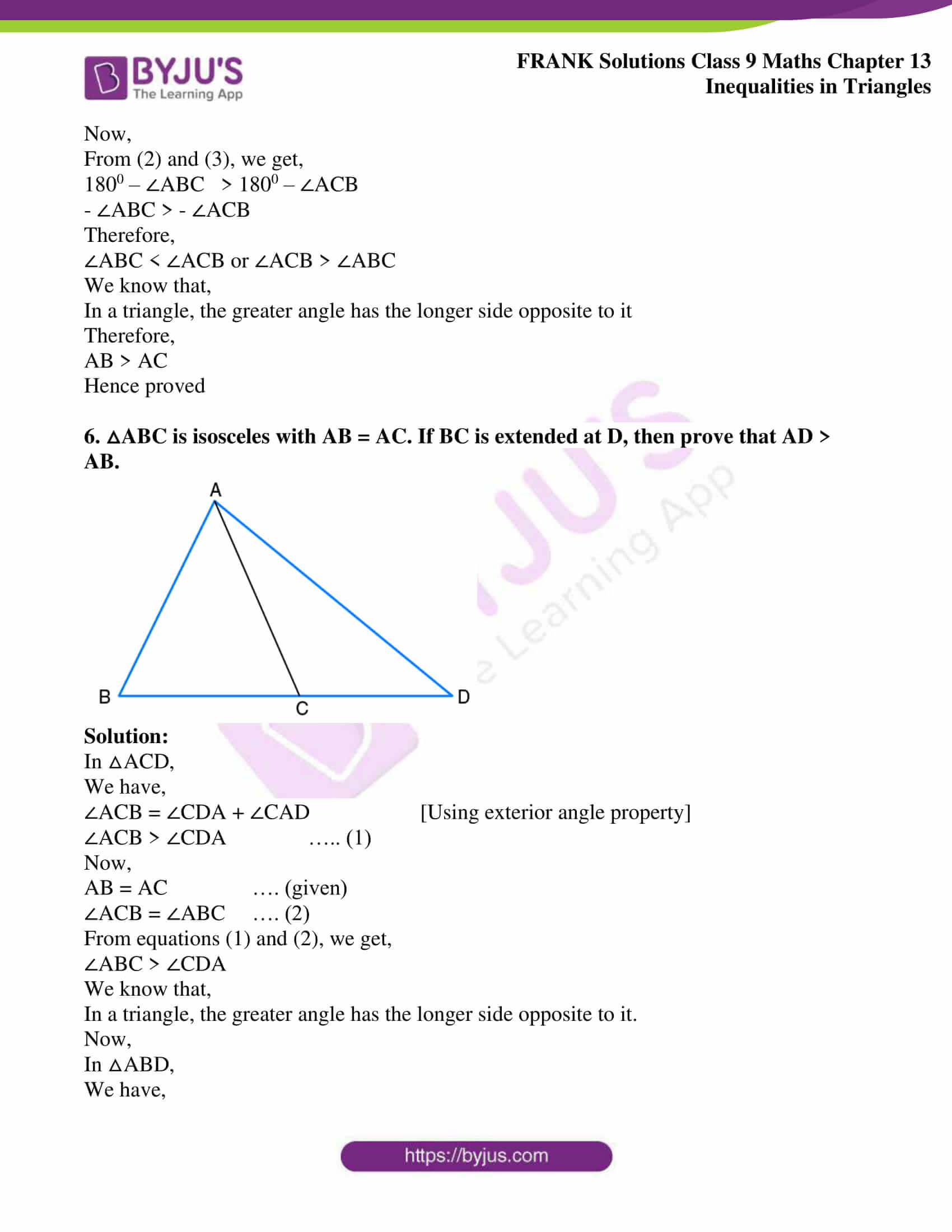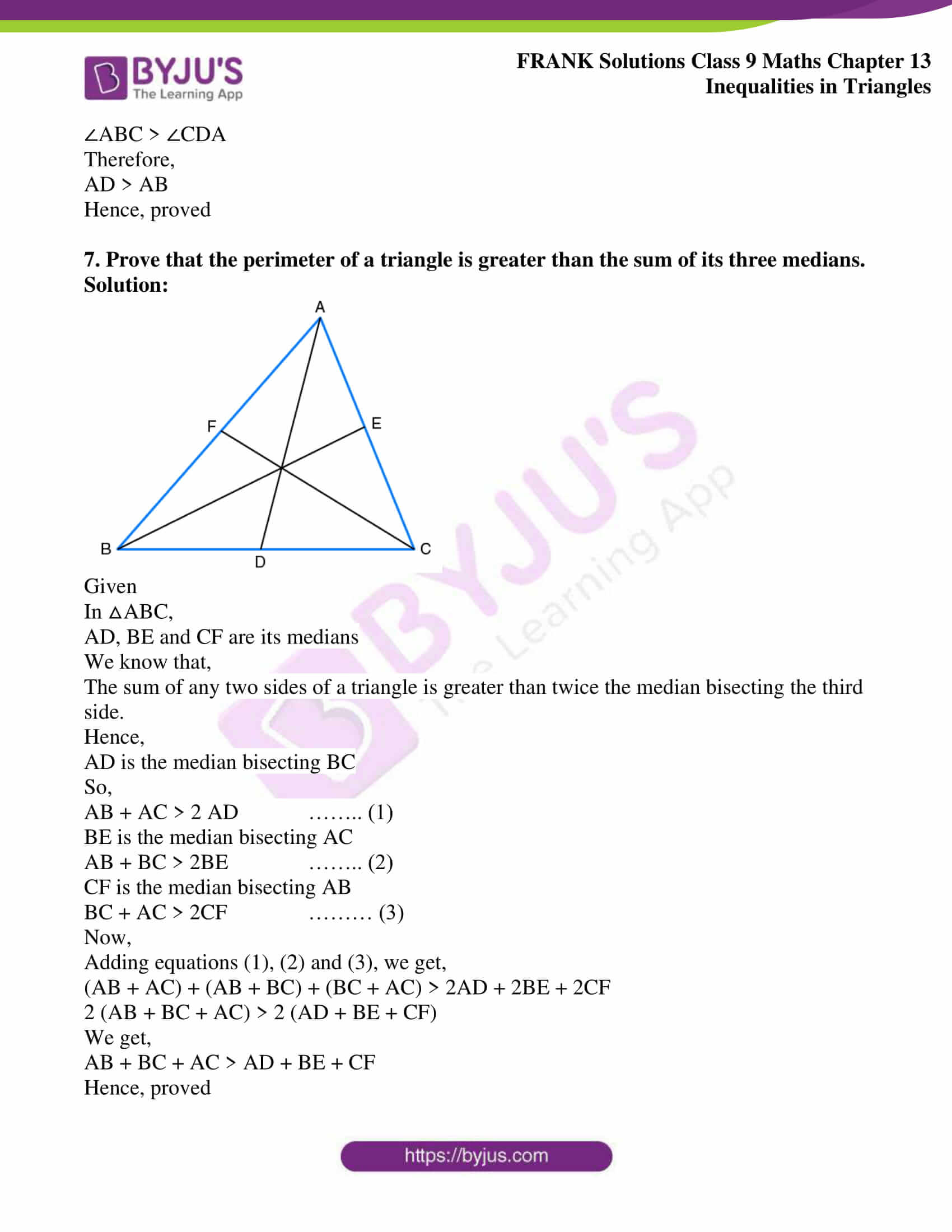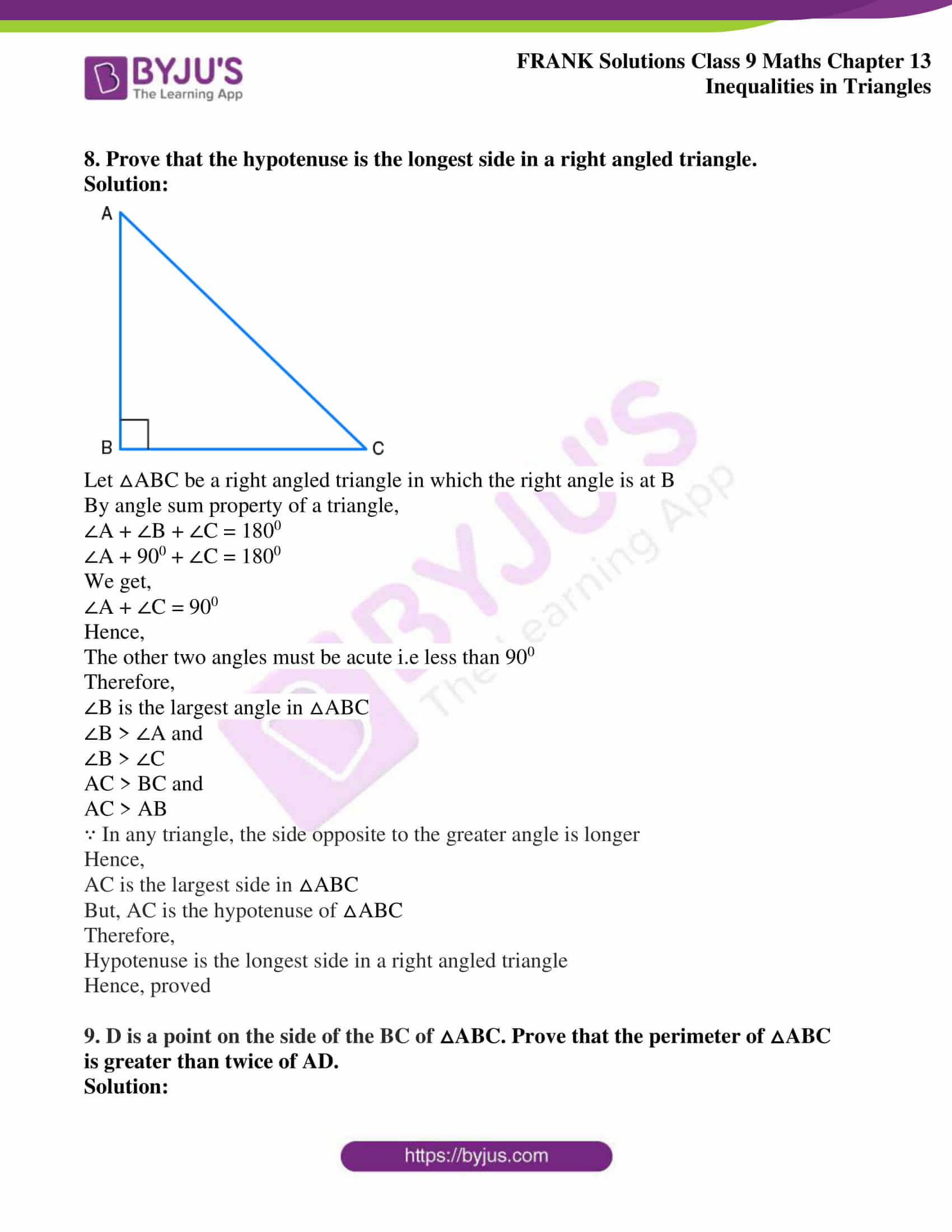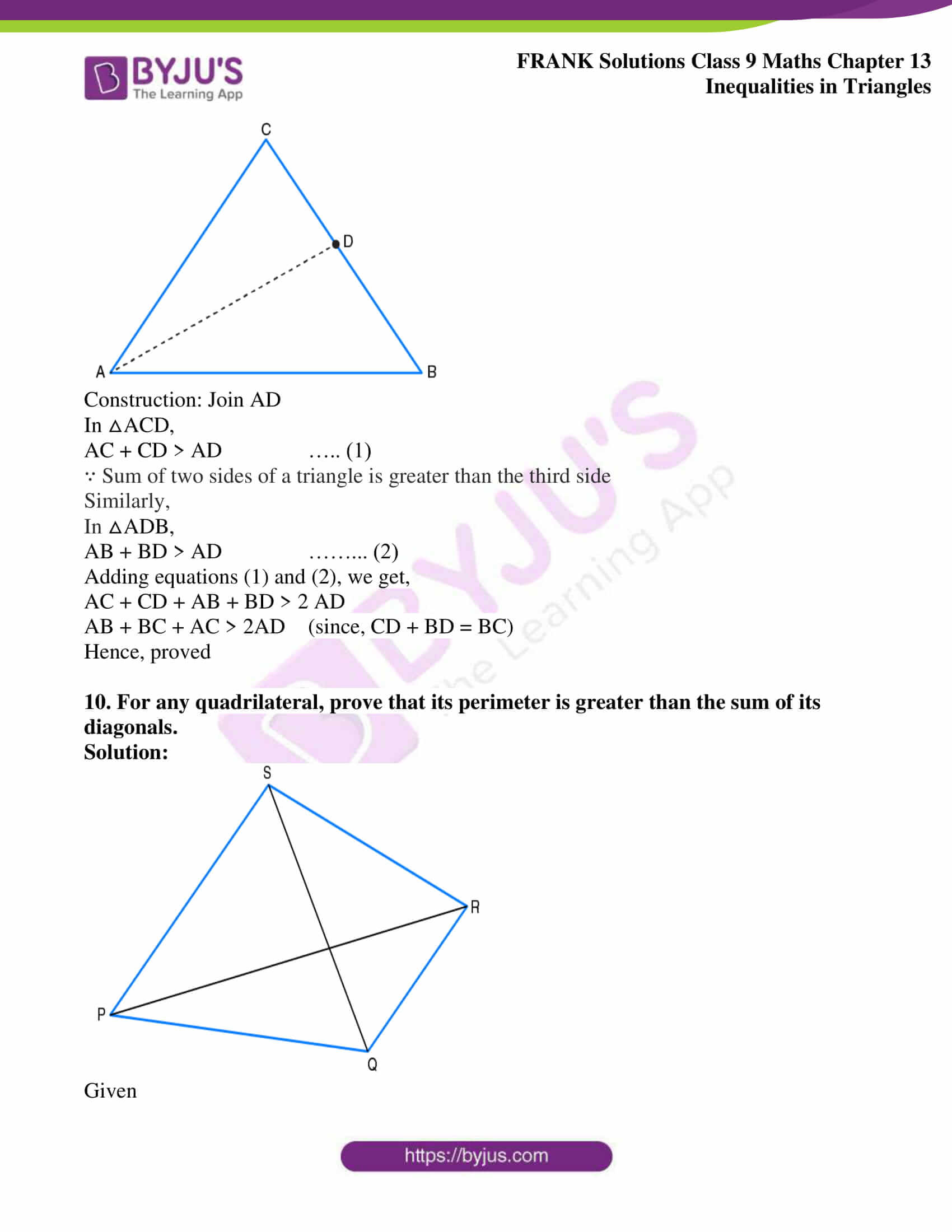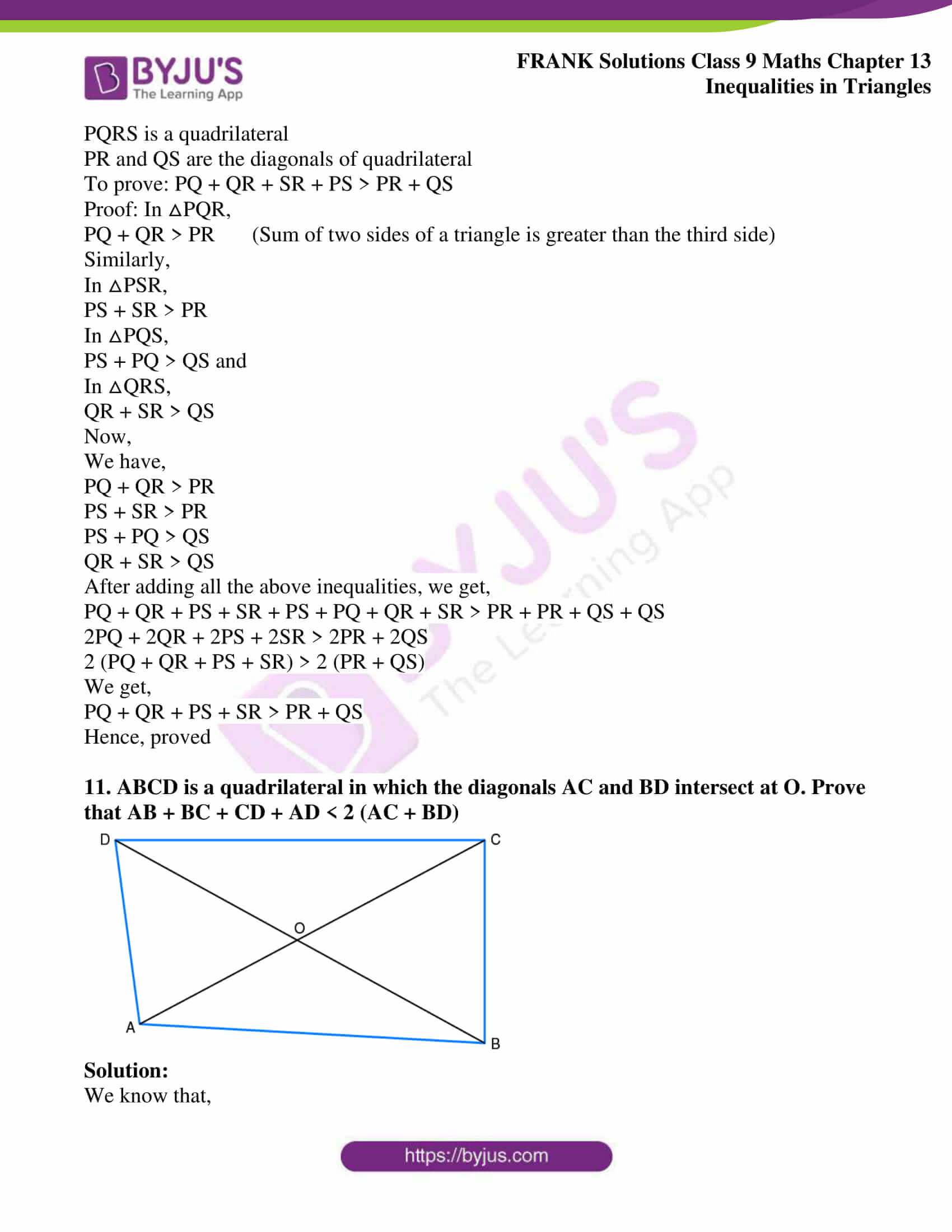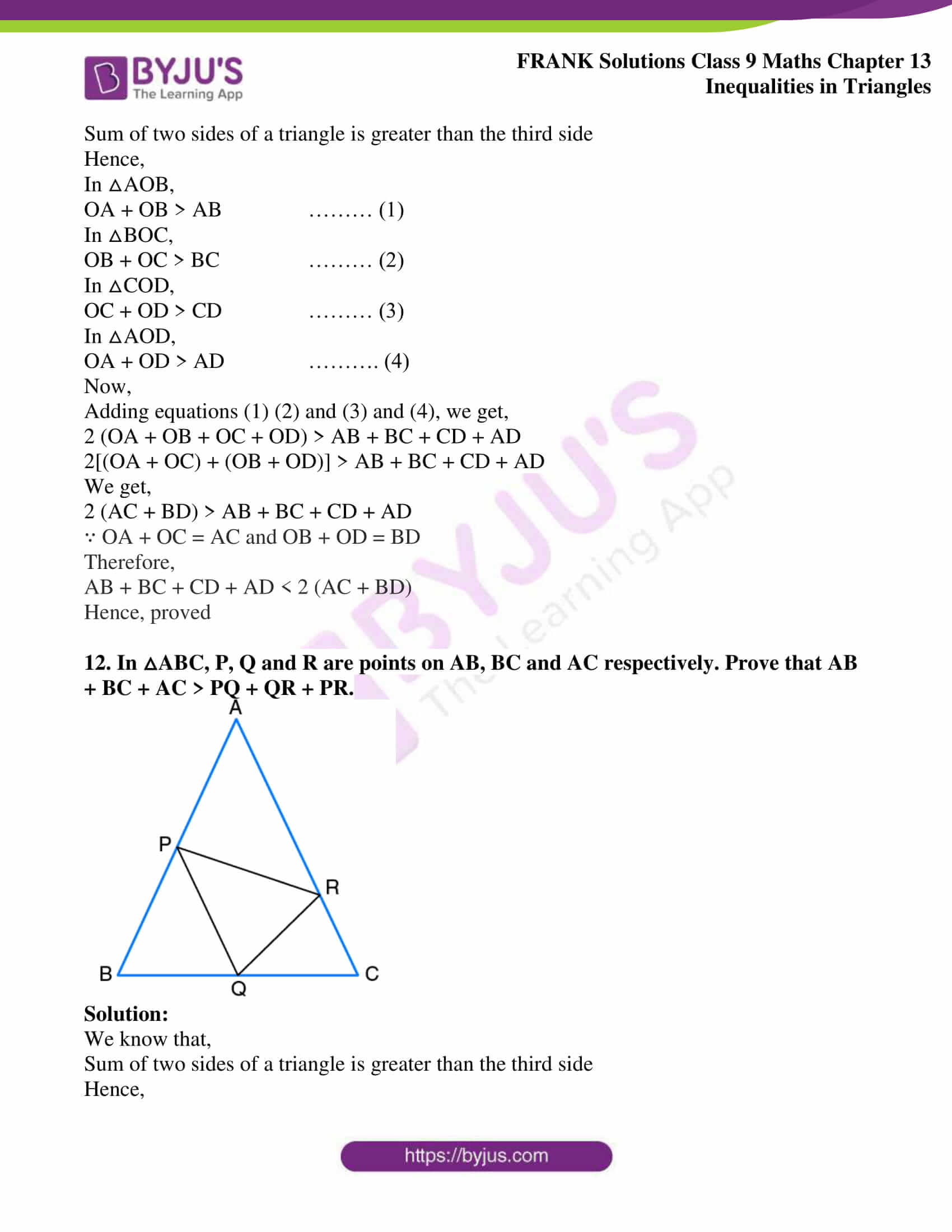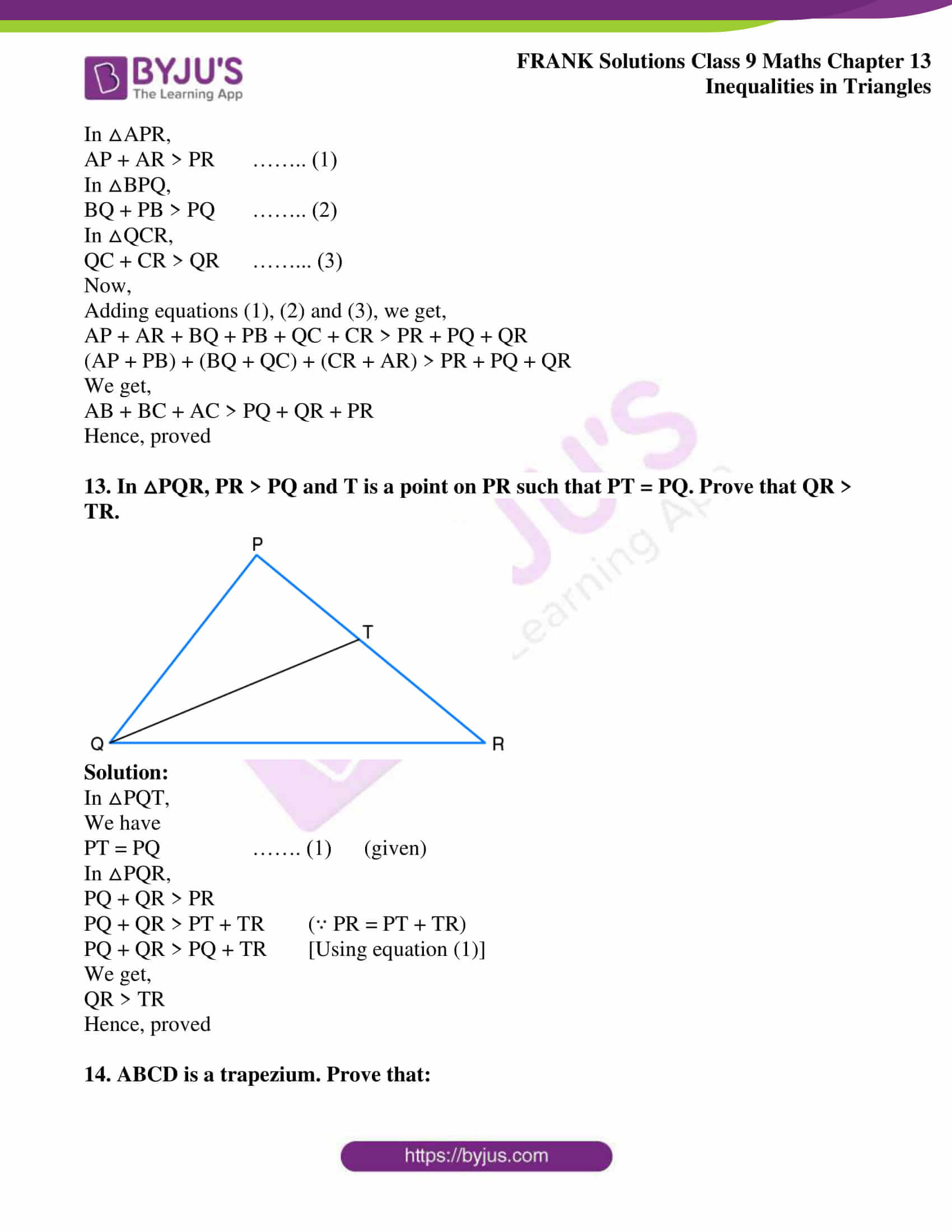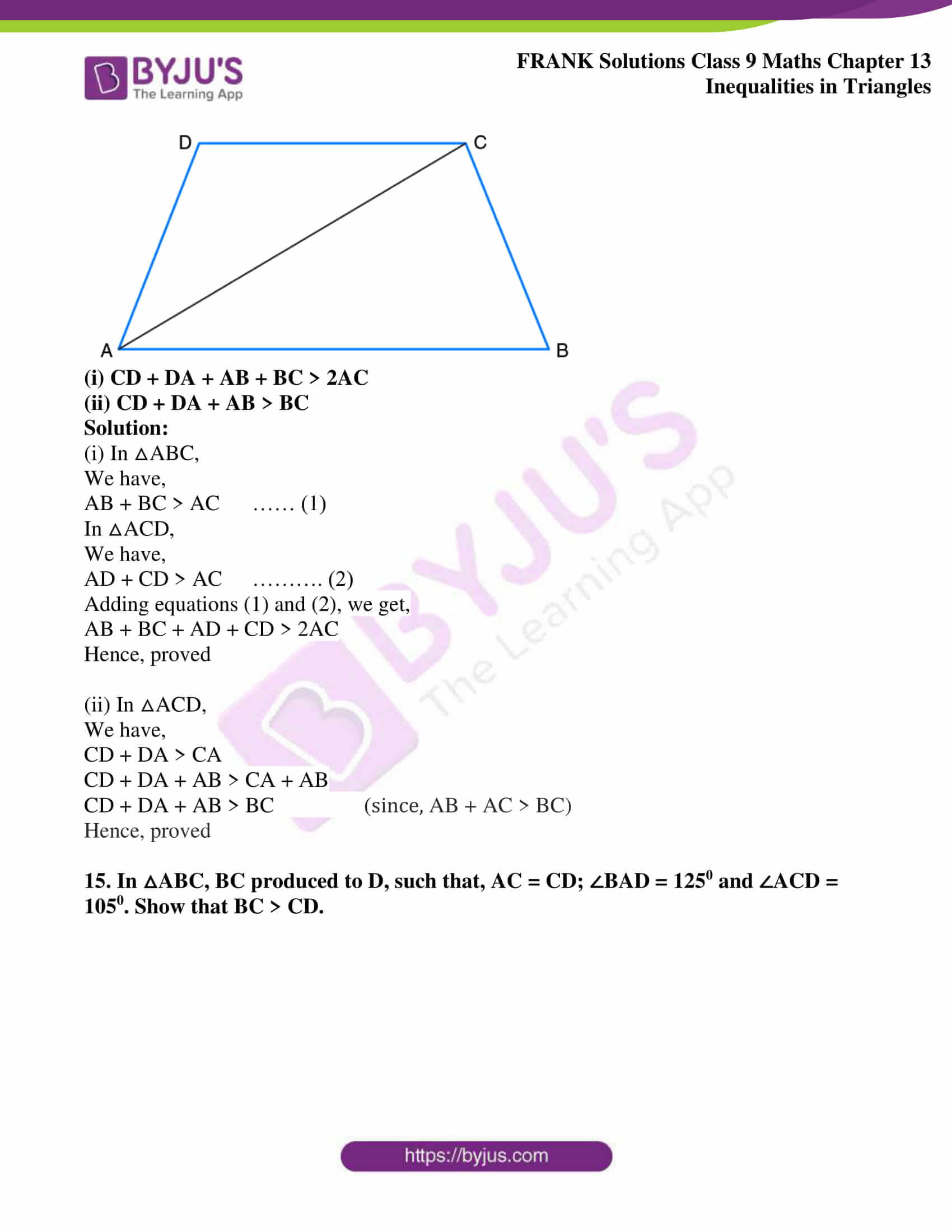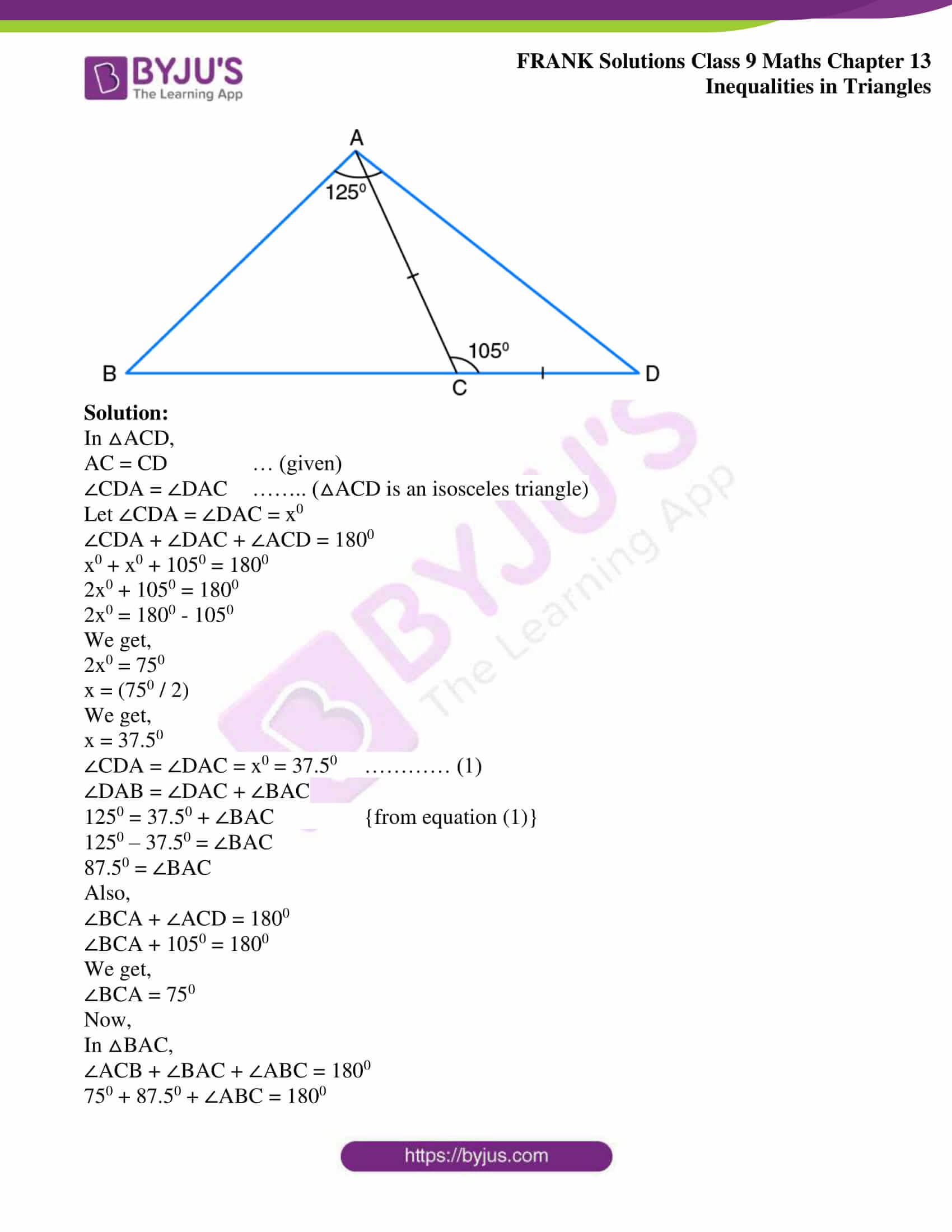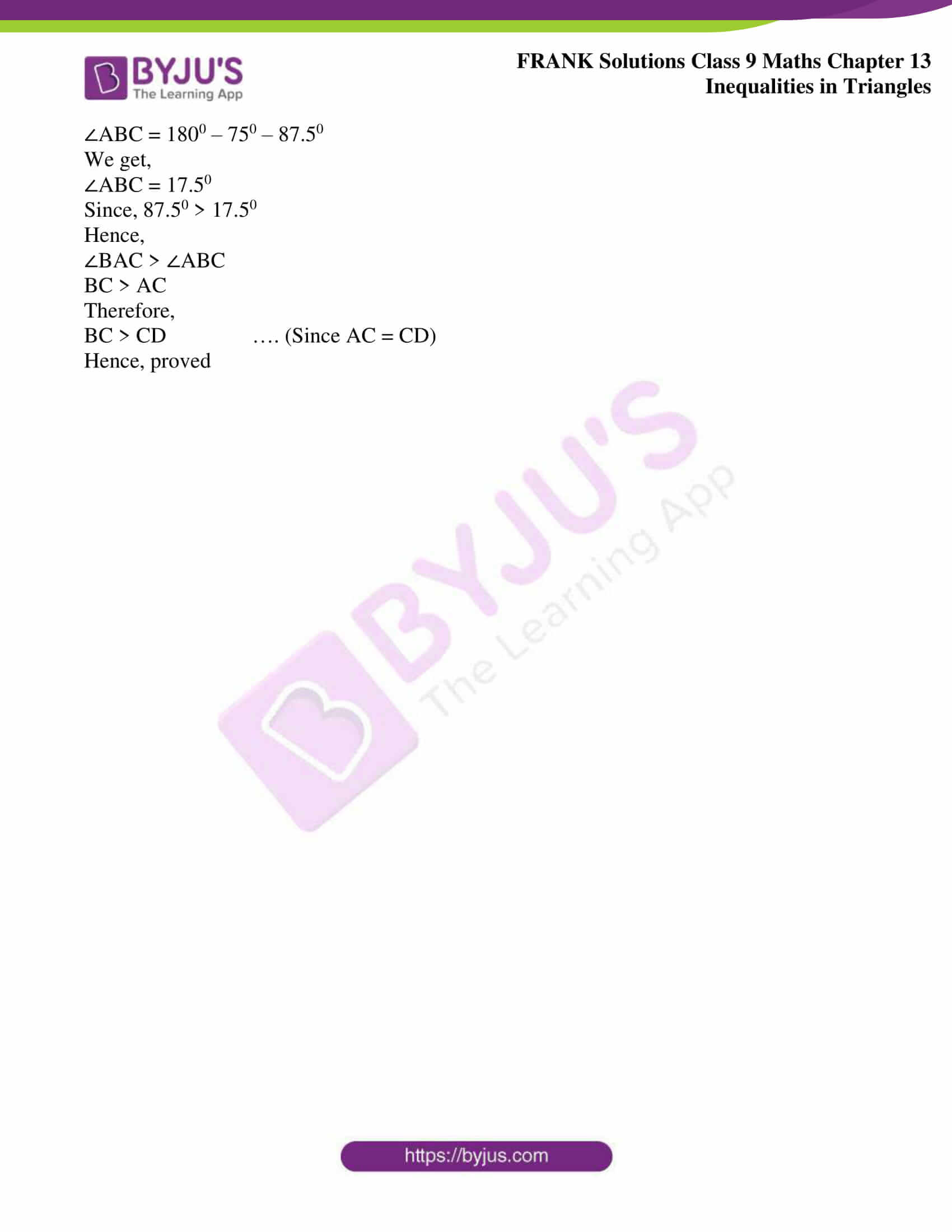## Access Frank Solutions for Class 9 Maths Chapter 13 Inequalities in Triangles

1. Name the greatest and the smallest sides in the following triangles:

(a) △ABC, ∠A = 560, ∠B = 640 and ∠C = 600

(b) △DEF, ∠D = 320, ∠E = 560 and ∠F = 920

(c) △XYZ, ∠X = 760, ∠Y = 840

Solution:

(a) In the given △ABC,

The greatest angle is ∠B and the opposite side to the ∠B is AC

Therefore,

The greatest side is AC

The smallest angle in the △ABC is ∠A and the opposite side to the ∠A is BC

Therefore,

The smallest side is BC

(b) In the given △DEF,

The greatest angle is ∠F and the opposite side to the ∠F is DE

Therefore,

The greatest side is DE

The smallest angle in the △DEF is ∠D and the opposite side to the ∠D is EF

Therefore,

The smallest side is EF

(c) In △XYZ,

∠X + ∠Y + ∠Z = 1800

760 + 840 + ∠Z = 1800

1600 + ∠Z = 1800

∠Z = 1800 – 1600

We get,

∠Z = 200

Therefore,

∠X = 760, ∠Y = 840, ∠Z = 200

In the given △XYZ,

The greatest angle is ∠Y and the opposite side to the ∠Y is XZ

Therefore,

The greatest side is XZ

The smallest angle in the △XYZ is ∠Z and the opposite side to the ∠Z is XY

Therefore,

The smallest side is XY

2. Arrange the sides of the following triangles in an ascending order:

(a) △ABC, ∠A = 450, ∠B = 650

(b) △DEF, ∠D = 380, ∠E = 580

Solution:

(a) In △ABC,

∠A + ∠B + ∠C = 1800

450 + 650 + ∠C = 1800

1100 + ∠C = 1800

∠C = 1800 – 1100

We get,

∠C = 700

Hence,

∠A = 450, ∠B = 650, ∠C = 700

450 < 650 < 700

Hence,

Ascending order of the angles in the given triangle is,

∠A < ∠B < ∠C

Therefore,

Ascending order of sides in triangle is,

BC, AC, AB

(b) In △DEF,

∠D + ∠E + ∠F = 1800

380 + 580 + ∠F = 1800

960 + ∠F = 1800

∠F = 1800 – 960

We get,

∠F = 840

Hence,

∠D = 380, ∠E = 580, ∠F = 840

380 < 580 < 840

Hence,

Ascending order of the angles in the given triangle is,

∠D < ∠E < ∠F

Therefore,

Ascending order of sides in triangle is,

EF, DF, DE

3. Name the smallest angle in each of these triangles:

(i) In △ABC, AB = 6.2 cm, BC = 5.6 cm and AC = 4.2 cm

(ii) In △PQR, PQ = 8.3 cm, QR = 5.4 cm and PR = 7.2 cm

(iii) In △XYZ, XY = 6.2 cm, XY = 6.8 cm and YZ = 5 cm

Solution:

(i) We know that,

In a triangle, the angle opposite to the smallest side is the smallest

In △ABC,

AC = 4.2 cm is the smallest side

Hence,

∠B is the smallest angle

(ii) We know that,

In a triangle, the angle opposite to the smallest side is the smallest

In △PQR,

QR = 5.4 cm is the smallest side

Hence,

∠P is the smallest angle

(iii) We know that,

In a triangle, the angle opposite to the smallest side is the smallest

In △XYZ,

YZ = 5 cm is the smallest side

Therefore,

∠X is the smallest angle

4. In a triangle ABC, BC = AC and ∠A = 350. Which is the smallest side of the triangle?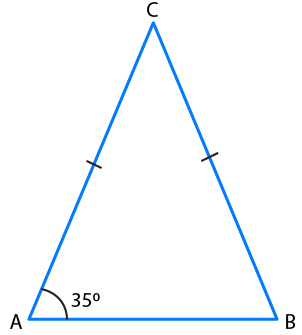Solution:

In △ABC,

BC = AC (given)

∠A = ∠B = 350 [angles opposite to the two equal sides are equal]

Let ∠C = x0

In △ABC,

∠A + ∠B + ∠C = 1800

350 + 350 + x0 = 1800

700 + x0 = 1800

x0 = 1800 – 700

We get,

x0 = 1100

Hence,

∠C = x0 = 1100

Therefore,

Angles in a triangle are ∠A = ∠B = 350 and ∠C = 1100

In △ABC,

The greatest angle is ∠C

Here, the smallest angles are ∠A and ∠B

Hence,

Smallest sides are BC and AC

5. In △ABC, the exterior ∠PBC > exterior ∠QCB. Prove that AB> AC.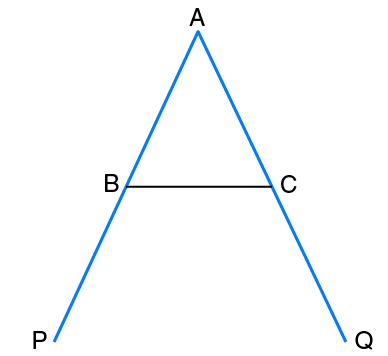Solution:

In the given triangle,

Given that, ∠PBC > ∠QCB ……. (1)

∠PBC + ∠ABC = 1800 (linear pair angles)

∠PBC = 1800 – ∠ABC …. (2)

Similarly,

∠QCB = 1800 – ∠ACB ……(3)

Now,

From (2) and (3), we get,

1800 – ∠ABC > 1800 – ∠ACB

– ∠ABC > – ∠ACB

Therefore,

∠ABC < ∠ACB or ∠ACB > ∠ABC

We know that,

In a triangle, the greater angle has the longer side opposite to it

Therefore,

AB > AC

Hence proved

6. △ABC is isosceles with AB = AC. If BC is extended at D, then prove that AD > AB.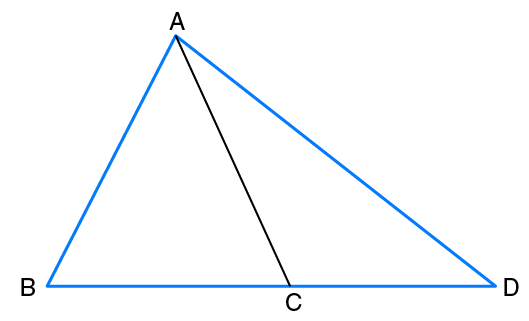Solution:

In △ACD,

We have,

∠ACB = ∠CDA + ∠CAD [Using exterior angle property]

∠ACB > ∠CDA ….. (1)

Now,

AB = AC …. (given)

∠ACB = ∠ABC …. (2)

From equations (1) and (2), we get,

∠ABC > ∠CDA

We know that,

In a triangle, the greater angle has the longer side opposite to it.

Now,

In △ABD,

We have,

∠ABC > ∠CDA

Therefore,

Hence, proved

7. Prove that the perimeter of a triangle is greater than the sum of its three medians.

Solution: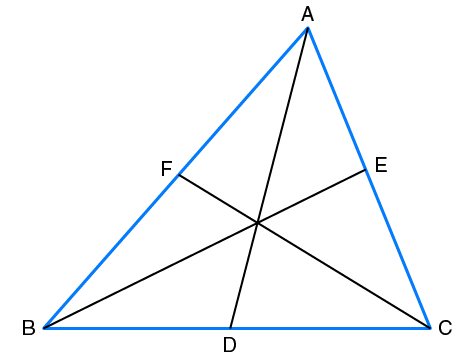Given

In △ABC,

AD, BE and CF are its medians

We know that,

The sum of any two sides of a triangle is greater than twice the median bisecting the third side.

Hence,

AD is the median bisecting BC

So,

AB + AC > 2 AD …….. (1)

BE is the median bisecting AC

AB + BC > 2BE …….. (2)

CF is the median bisecting AB

BC + AC > 2CF ……… (3)

Now,

Adding equations (1), (2) and (3), we get,

(AB + AC) + (AB + BC) + (BC + AC) > 2AD + 2BE + 2CF

2 (AB + BC + AC) > 2 (AD + BE + CF)

We get,

AB + BC + AC > AD + BE + CF

Hence, proved

8. Prove that the hypotenuse is the longest side in a right angled triangle.

Solution: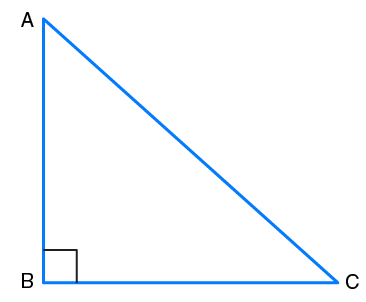Let △ABC be a right angled triangle in which the right angle is at B

By angle sum property of a triangle,

∠A + ∠B + ∠C = 1800

∠A + 900 + ∠C = 1800

We get,

∠A + ∠C = 900

Hence,

The other two angles must be acute i.e less than 900

Therefore,

∠B is the largest angle in △ABC

∠B > ∠A and

∠B > ∠C

AC > BC and

AC > AB

∵ In any triangle, the side opposite to the greater angle is longer

Hence,

AC is the largest side in △ABC

But, AC is the hypotenuse of △ABC

Therefore,

Hypotenuse is the longest side in a right angled triangle

Hence, proved

9. D is a point on the side of the BC of △ABC. Prove that the perimeter of △ABC

is greater than twice of AD.

Solution: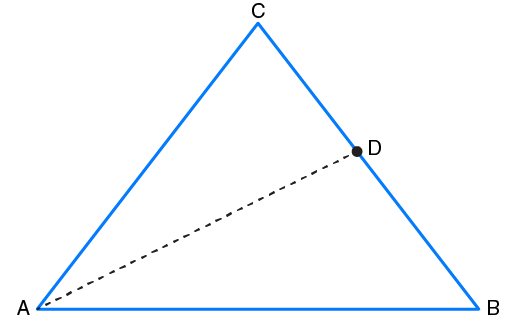In △ACD,

AC + CD > AD ….. (1)

∵ Sum of two sides of a triangle is greater than the third side

Similarly,

AB + BD > AD ……… (2)

Adding equations (1) and (2), we get,

AC + CD + AB + BD > 2 AD

AB + BC + AC > 2AD (since, CD + BD = BC)

Hence, proved

10. For any quadrilateral, prove that its perimeter is greater than the sum of its diagonals.

Solution: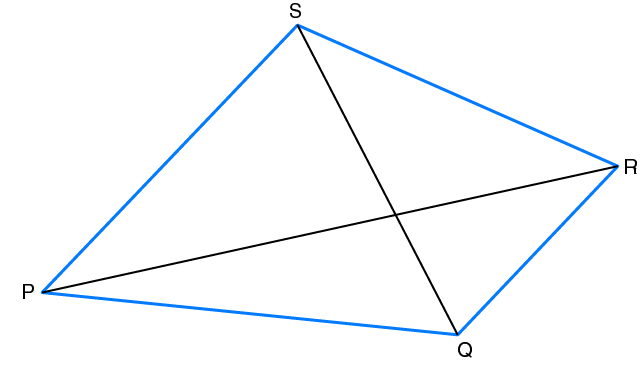Given

PR and QS are the diagonals of quadrilateral

To prove: PQ + QR + SR + PS > PR + QS

Proof: In △PQR,

PQ + QR > PR (Sum of two sides of a triangle is greater than the third side)

Similarly,

In △PSR,

PS + SR > PR

In △PQS,

PS + PQ > QS and

In △QRS,

QR + SR > QS

Now,

We have,

PQ + QR > PR

PS + SR > PR

PS + PQ > QS

QR + SR > QS

After adding all the above inequalities, we get,

PQ + QR + PS + SR + PS + PQ + QR + SR > PR + PR + QS + QS

2PQ + 2QR + 2PS + 2SR > 2PR + 2QS

2 (PQ + QR + PS + SR) > 2 (PR + QS)

We get,

PQ + QR + PS + SR > PR + QS

Hence, proved

11. ABCD is a quadrilateral in which the diagonals AC and BD intersect at O. Prove that AB + BC + CD + AD < 2 (AC + BD)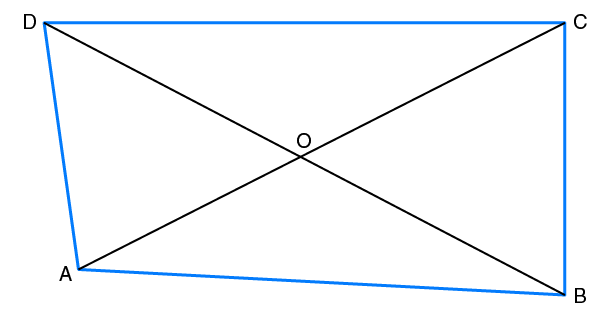Solution:

We know that,

Sum of two sides of a triangle is greater than the third side

Hence,

In △AOB,

OA + OB > AB ……… (1)

In △BOC,

OB + OC > BC ……… (2)

In △COD,

OC + OD > CD ……… (3)

In △AOD,

OA + OD > AD ………. (4)

Now,

Adding equations (1) (2) and (3) and (4), we get,

2 (OA + OB + OC + OD) > AB + BC + CD + AD

2[(OA + OC) + (OB + OD)] > AB + BC + CD + AD

We get,

2 (AC + BD) > AB + BC + CD + AD

∵ OA + OC = AC and OB + OD = BD

Therefore,

AB + BC + CD + AD < 2 (AC + BD)

Hence, proved

12. In △ABC, P, Q and R are points on AB, BC and AC respectively. Prove that AB + BC + AC > PQ + QR + PR.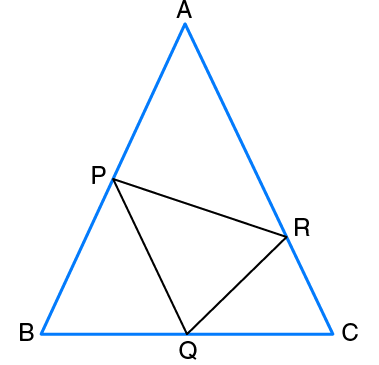Solution:

We know that,

Sum of two sides of a triangle is greater than the third side

Hence,

In △APR,

AP + AR > PR …….. (1)

In △BPQ,

BQ + PB > PQ …….. (2)

In △QCR,

QC + CR > QR ……… (3)

Now,

Adding equations (1), (2) and (3), we get,

AP + AR + BQ + PB + QC + CR > PR + PQ + QR

(AP + PB) + (BQ + QC) + (CR + AR) > PR + PQ + QR

We get,

AB + BC + AC > PQ + QR + PR

Hence, proved

13. In △PQR, PR > PQ and T is a point on PR such that PT = PQ. Prove that QR > TR.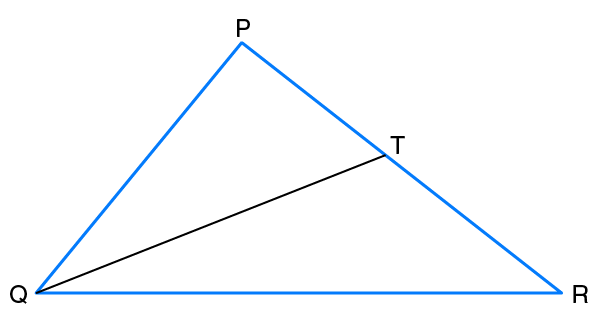Solution:

In △PQT,

We have

PT = PQ ……. (1) (given)

In △PQR,

PQ + QR > PR

PQ + QR > PT + TR (∵ PR = PT + TR)

PQ + QR > PQ + TR [Using equation (1)]

We get,

QR > TR

Hence, proved

14. ABCD is a trapezium. Prove that: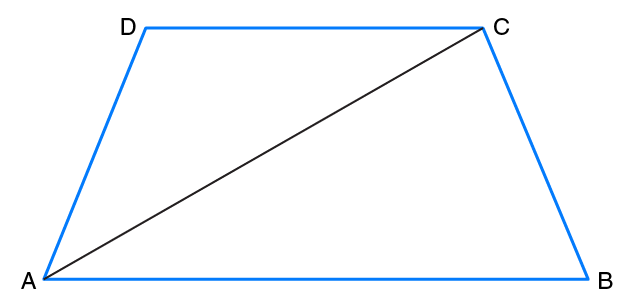(i) CD + DA + AB + BC > 2AC

(ii) CD + DA + AB > BC

Solution:

(i) In △ABC,

We have,

AB + BC > AC …… (1)

In △ACD,

We have,

AD + CD > AC ………. (2)

Adding equations (1) and (2), we get,

AB + BC + AD + CD > 2AC

Hence, proved

(ii) In △ACD,

We have,

CD + DA > CA

CD + DA + AB > CA + AB

CD + DA + AB > BC (since, AB + AC > BC)

Hence, proved

15. In △ABC, BC produced to D, such that, AC = CD; ∠BAD = 1250 and ∠ACD = 1050. Show that BC > CD.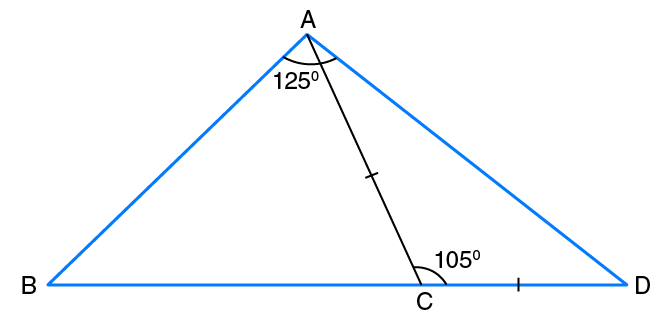Solution:

In △ACD,

AC = CD … (given)

∠CDA = ∠DAC …….. (△ACD is an isosceles triangle)

Let ∠CDA = ∠DAC = x0

∠CDA + ∠DAC + ∠ACD = 1800

x0 + x0 + 1050 = 1800

2x0 + 1050 = 1800

2x0 = 1800 – 1050

We get,

2x0 = 750

x = (750 / 2)

We get,

x = 37.50

∠CDA = ∠DAC = x0 = 37.50 ………… (1)

∠DAB = ∠DAC + ∠BAC

1250 = 37.50 + ∠BAC {from equation (1)}

1250 – 37.50 = ∠BAC

87.50 = ∠BAC

Also,

∠BCA + ∠ACD = 1800

∠BCA + 1050 = 1800

We get,

∠BCA = 750

Now,

In △BAC,

∠ACB + ∠BAC + ∠ABC = 1800

750 + 87.50 + ∠ABC = 1800

∠ABC = 1800 – 750 – 87.50

We get,

∠ABC = 17.50

Since, 87.50 > 17.50

Hence,

∠BAC > ∠ABC

BC > AC

Therefore,

BC > CD …. (Since AC = CD)

Hence, proved